Maths-
General
Easy

Question

# In a fraction, if the numerator is increased by 2 and the denominator is decreased by 3, then the fraction becomes 1. Instead, if the numerator is decreased by 2 and denominator is increased by 3, then the fraction becomes 3/ 8. Find the fraction.Hint:

## The correct answer is: 8/13

### Ans :- 8/13 is the value of fraction .Explanation :-Step 1:- Frame the equation based on given conditions.let the  numerator of fraction be x and denominator of fraction is yif the numerator is increased by 2 and the denominator is decreased by 3, then the fraction becomes 1We get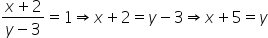— Eq1If the numerator is decreased by 2 and denominator is increased by 3, then the fraction becomes 3/ 8.We get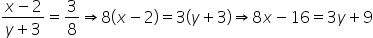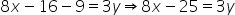— Eq2Step 2:- eliminate the y and find xDoing Eq2 -3(Eq1) to eliminate y .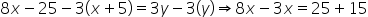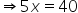∴x = 8 Step 3:- find y by substituting the value of x in eq1.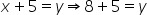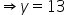∴y = 13 We know the fraction is x/y =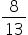#### With Turito Foundation.#### Get an Expert Advice From Turito.